# SSC Quantitative Aptitude Practice Questions (Day-2)

Dear Aspirants, Here we have given the Important SSC CGL Exams 2018 Practice Test Papers. Candidates, those who are preparing for SSC CGL Exams 2018 can practice these questions to get more confidence to Crack SSC CGL Exams 2018 Examination.

[WpProQuiz 4011]

1) The average age of 50 students and the principal is 25 years. When the principal’s age is excluded, the average age decreases by 1 year. What is the age (in years) of the principal?

a) 65

b) 75

c) 74

2) How many liters of water should be added to a 30 liter mixture of milk and water containing milk and water in the ratio of 7: 3 such that the resultant mixture has 50% water in it?

a) 12 liters

b) 13 liters

c) 11 liters

d) 15 liters

3) The price of tea is reduced by 25% but in spite of the decrease, Aakash ends up increasing his expenditure on tea by 20%. What is the percentage change in his monthly consumption of tea?

a) + 60

b) – 10

c) + 33.33

d) +50

4) A wheat merchant makes a profit of 10% by selling at Rs.2200 per quintal. If he sells the wheat at Rs.2250 per quintal, what is his profit percent on the whole investment?

a) 6

b) 6.66

c) 12.5

d) 8

5) The cost of production of a laptop is Rs.9000 divided between material, labour and overheads expenses in the ratio 3:4:2. If the laptop set is marked at a price that gives a 20% profit on the component of price accounted for by labour, what is the marked price of the set?

a) Rs.9800

b) Rs. 10,800

c) Rs, 9600

d) Rs, 10,200

6) If after adding simple interest on an amount for 3 years at 5% per annum the sum becomes Rs.3540, what is the compound interest on the same sum at double rate and for the same period of time?

a) Rs. 331

b) Rs. 993

c) Rs. 662

d) None of these

7) The proportion of milk and water in 3 samples is 2:1, 3 : 2 and 5 : 3. A mixture comprising of equal quantities of all 3 samples is made. The proportion of milk and water in the mixture is:

a) 2 : 1

b) 5 : 1

c) 99 : 61

d) 227 : 133

8) A, B, C and D can do a piece of work in 10, 20, 40 and 80 hours, respectively. A starts the work, B joins when A leaves after 1/4th of the work is done, C replaces B after half of the work is completed and D replaces C after 3/4th of the work. D completes the work. Find the time (in hours) after which the work gets completed.

a) 36

b) 37.5

c) 37

d) 38.5

9) Two persons Akram and Vakram start moving towards each other at the same time from two points 180m apart. Time taken by Akram and Vakram to reach the opposite end after meeting in 8 seconds and 18 seconds, respectively. What is the speed of Akram?

a) 6 m/s

b) 8 m/s

c) 9 m/s

d) 7.5 m/s

10) An article is listed at Rs. 65. A customer bought this article for Rs 56.16 and got two successive discounts of which the first one is 10%. The other rate of discount of this scheme that was allowed by the shopkeeper was

a) 3%

b) 4%

c) 6%

d) 2%

11) p/x + q/y = m and q/x + p/y = n what is the value of x/y?

a) (np + mq)/ mp+nq

b) (np + mq)/ (mp – nq)

c) (np – mp)/ (mp – np)

d) (np – mp) / (mp + nq)

12) If a2 – by – cz = 0 ax – b2 + cz = 0, then find the value of

a) a + b + c

b) 3

c) 1

d) 0

13) If x = 21/3 + 2 1/3, then the value of 2x2 – 6x – 5 is

a) 0

b) 1

c) 2

d) 3

14) The value of sin35°/ cos 55° – cos 55° / sin 35° + 2sin 30 ° is

a) -1

b) 1

c) 2

d) 3

15) Find the value of k, for which the system of equations 3x – ky – 20 = 0 and 6x – 10y + 40 = 0 has no solution, is:

a) 1

b) 2

c) 5

d) 10

Let the average age of students = S years, and the age of principal = P years

According to the question,

(50×S + P) / 51 = 25

After excluding the principle’s age, the new average age of all students = S = 25 – 1 = 24

S = 24

(50×24 + P) / 51 = 25

1200 + P = 25 × 51

P = 1275 – 1200 = 75

30 liters of mixture has milk and water in the ratio 7 : 3, i.e. the solution has 21 liters of milk and 9 liters of water.

When you add more water, the amount of milk in the mixture remains constant at 21 liters.

In the first case, before addition of further water, 21 liters of milk accounts for 70% by volume.

After water is added, the new mixture contains 50% milk and 50% water.

Therefore, the 21litres of milk accounts for 50% by volume. Hence, 100% volume = (21/0.5) = 42 liters.

We started with 30 liters and ended up with 42 liters. Therefore, 12 liters of water has to be added.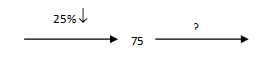We have assumed initial expenditure to be 100, in the above figure. Then the final expenditure is 120.

The percentage change in consumption can be seen to be (45* 100)/75 = 60%

Here, (110 CP/100) = 2200 or CP = 2000

At a selling price of Rs.2250 the profit percent = 250 × 100/2000 = 12.5

The labour price accounts for Rs.4000.

Since the profit percentage gives a 20% profit on this component, i.e. Rs.800.

Hence, the marked price is Rs.9800.

Let the principal sum be Rs. x, then

x + 0.15x = 3540, or x = Rs.3000.

The compound interest on Rs.3000 at the rate of 10% per annum for 3 years would be given by:

Interest for the first year = 10% of 3000 = Rs.300

Interest for the second year = 10% of (3000 + 300) = 300 + 30 = Rs.330

Interest for the third year = 10% of (3300 + 330) = 330 + 33 = Rs.363

Thus, total interest = 300 + 330 + 363 = Rs.993

Proportion of milk in 3 samples = 2/3, 3/5, 5/8

Proportion of water in 3 samples = 1/3, 2/5, 3/8.

Since equal quantities are taken, so

Total proportion of milk = 2/3 + 3/5 + 5/8 = 227/120

Total proportion of water = 1/3 + 2/5 + 3/8 = 133/120

Proportion of milk and water in the solution = 227: 133

Let the total units of work be 80 (LCM of 10, 20, 40, and 80) units.

Efficiencies per day

A = 80/10 = 8,

B = 80/20 = 4,

C = 80/40 = 2,

D = 80/80 = 1,

According to the question, 20 units is done by A alone, 20 units is done by B, 20 units is done by C and 20 units is done by D. Hence, the required time = 20/8 + 20/4 + 20/2 + 20/1 = 37.5 hours.

Time taken after meeting is 8 seconds and 18 seconds.

Hence time taken to meet would be the square root of (8 × 18) => 12

Hence, time taken by Akram = 12 + 8 = 20 sec.

Speed of Vakram = 180/20 = 9 m/sec

Price of the article after first discount = 65 – 6.5 = Rs. 58.5

Therefore, the second discount = [(58.5 – 56.16)/ 58.5] * 100 = 4%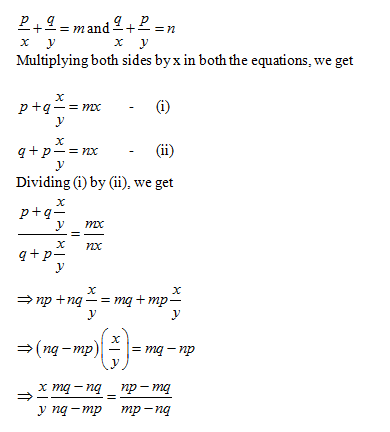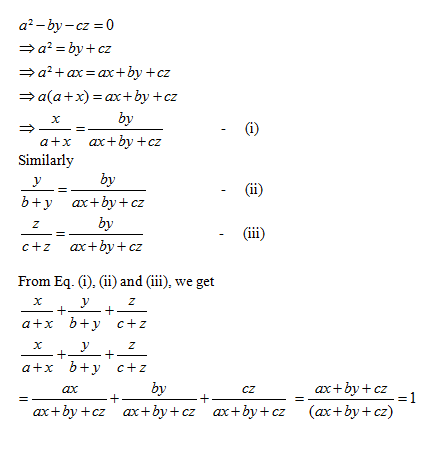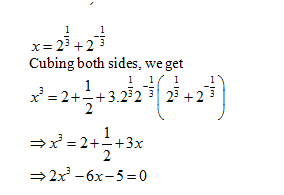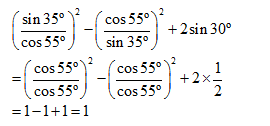3x – ky – 20 = 0

6x – 10y + 40 = 0

For no solution,

3/6 = k/10 ≠−20 / 40

K = 5